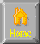```
OPTIMIZATION THEORY
++SIMPLEX METHOD

|
|
|\
| \
|  \
|   \
|-___\__
|     \ ---___________
|      \
+------------------------
/\
Polytope

B+W < 350
2B+W< 600
B+3W< 900

(1947 George Dantzig)
LP (Lin Prog) Solution always @ corner points on Boundary of Polytope
Algebraic Topology - polytope (Feasible region) = "SimpleX"

Simplex Method
1. Find a vertex in polytope & find value  @ this pt
2. Examine each edge attached to this vertex
3. Move alone edge w/ best improvement of criterion
4. Repeat 2-3

Graph Theory (1736 Leonhard Euler)
Nodes
a. Beginning - odd incident degree
b. End - odd incident degree
c. Intermediate Node - even incident degree
.--X--.
/ _/    \
|_/       |
X_________X
|\        |
\ \_      /
\_ \   _/
--X--

Min Cut-Max Flow Theorem
V* = Max steady state flow of s& t (source- sink)
c(P*,~P*) = capacity of minimum cut
-> V* = c(P*,~P*)
cut = node sets P & ~P,  P w/ source node, ~P w/ sink node
Capacity = c(P,~P) = sum of edges w/ one node in P and the other endpt in ~P

__
/  \
->|  |-
/  \__/ \
/    |\   \
/     ||    \
/      \|     \
__ /       __      \  __
/  \       /  \       /  \
|  | ----> |  |       |  |
\__/       \__/       \__/
\       |
\      |
\     \
\    __
\  /  \
->|  |
\__/

Gradient Method/Routing Networks
1. 1931 W. Gropius (SAR/OSR) Housing Block
P=alx/b
A=l(a+b)
I=3x/s
A/P = b(aI+3x) / alx
OSR = bsl/alx = bs/ax = sl/P
SAR -b/x = A/P -  b/x = sl/P = OSR

2. HILL CLIMB
Move in direction of steepest ascent/Gradient
Abraham wald- dynamic programming
3. Network routing (c.1950 R. Bellman)
Optimal value func - minimal cost tour city k to city N
optimal policy func j*(k) next city if at city k
4. Money allocation
principle of optimality

I3(X) = Max return of 3 investments w/ capital \$X
I3(X)=max(0.xc.X)[g3(xc)+I2(X-xc)]
g1(y)=return of investment 1 w/ \$y

```Home